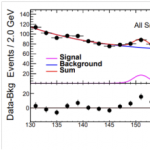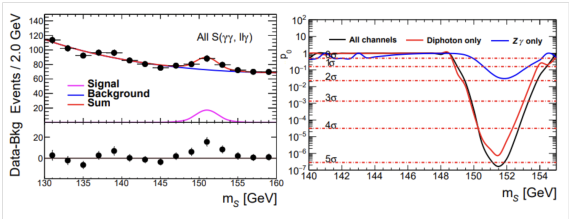# Paper of the month:  Accumulating Evidence for the Associate Production of a Neutral Scalar with Mass around 151 GeV#### October 20, 2021 by RebecaBV

Abstract: To establish the discovery of a new phenomenon (for example, a new particle or interaction) an experiment must reach a certain level of statistical significance.

More precisely, the probability of deviations from the null hypothesis (in which the new particle or interaction is not present) equal or greater than the observed deviation must fall below a certain value.

This probability is known as the p-value. In the particle physics literature, the p-value is often converted to a significance Z, defined as the number of standard deviations giving the same probability when integrating the standard Gaussian distribution from Z to ∞. Values of the p-value below 2.87×107 (corresponding to a 5σ significance) usually define a discovery.Attached “Accumulating Evidence for the Associate Production of a Neutral Scalar with Mass around 151 GeV” report by Patrick Bolton (INFN ESR) and Arturo de Giorgi (IFT-UAM ESR).